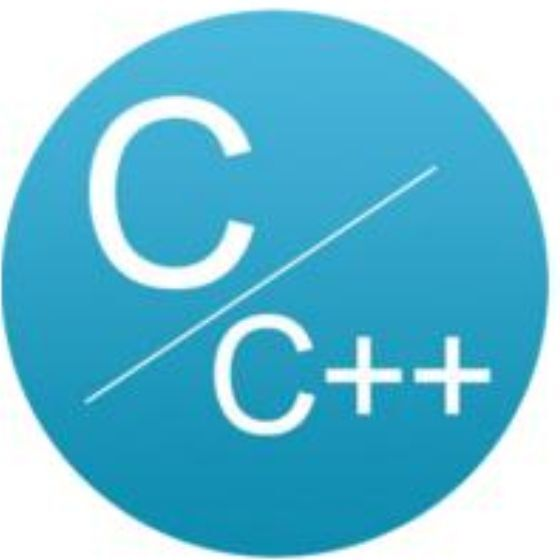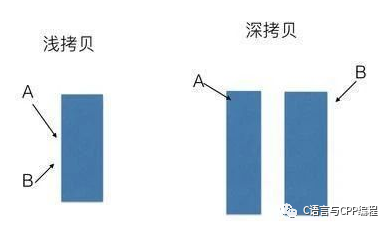# 深拷贝与浅拷贝到底是什么C语言与CPP编程

﻿

﻿﻿

#include <iostream>using namespace std;//20200430 公众号：C语言与CPP编程class CopyDemo{public:  CopyDemo(int pa,char *cstr)  //构造函数，两个参数  {     this->a = pa;     this->str = new char; //指针数组，动态的用new在堆上分配存储空间     strcpy(this->str,cstr);    //拷贝过来  }//没写，C++会自动帮忙写一个复制构造函数，浅拷贝只复制指针,如下注释部分  //CopyDemo(CopyDemo& obj)    //{  //   this->a = obj.a;  //  this->str = obj.str; //这里是浅复制会出问题，要深复制  //}  CopyDemo(CopyDemo& obj)  //一般数据成员有指针要自己写复制构造函数，如下  {     this->a = obj.a;    // this->str = obj.str; //这里是浅复制会出问题，要深复制     this->str = new char;//应该这样写     if(str != 0)        strcpy(this->str,obj.str); //如果成功，把内容复制过来  }  ~CopyDemo()  //析构函数  {     delete str;  }public:     int a;  //定义一个整型的数据成员     char *str; //字符串指针};int main(){  CopyDemo A(100,"hello!!!");  CopyDemo B = A;  //复制构造函数，把A的10和hello!!!复制给B  cout <<"A:"<< A.a << "," <<A.str << endl;  //输出A:100，hello!!!  cout <<"B:"<< B.a << "," <<B.str << endl;  //输出B:100，hello!!!  //修改后,发现A,B都被改变，原因就是浅复制，A,B指针指向同一地方，修改后都改变  B.a = 80;  B.str = 'k';  cout <<"A:"<< A.a << "," <<A.str << endl;  //输出A:100，kello!!!  cout <<"B:"<< B.a << "," <<B.str << endl;  //输出B:80，kello!!!  return 0;}

﻿﻿## 评论